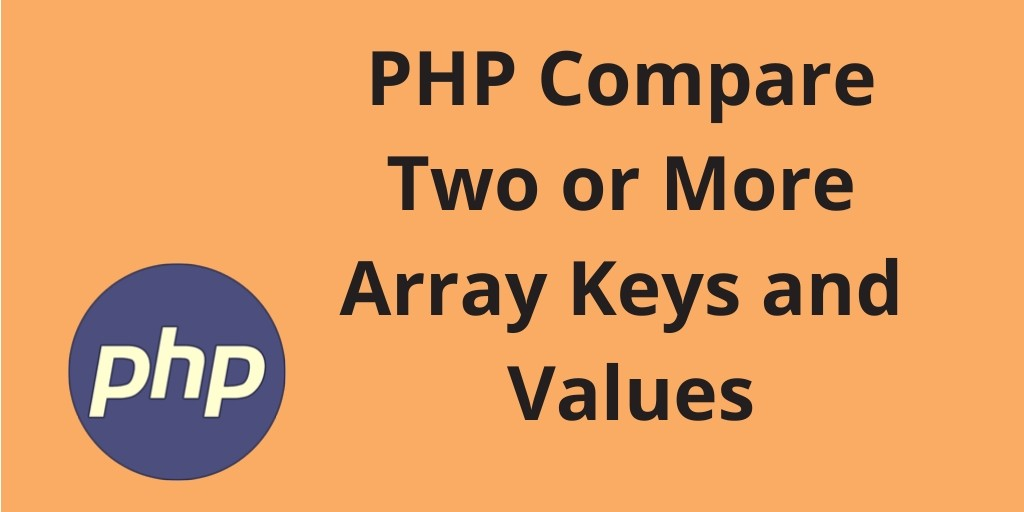Php 09-Aug-2021

### Compare Arrays Keys and Values | PHP array_diff_assoc()

PHP compares arrays of keys and values. In this tutorial, you will learn how to PHP compare two or more array keys and values and find the difference between two or more array using the PHP array_diff_assoc() function.

In addition, you will learn about the PHP array_diff_assoc () function, definition, syntax, parameters, and see various examples.

## PHP Compare Two or More Arrays Keys and Values

### PHP array_diff_assoc()

Definition: array_diff_assoc() function of in-built PHP. Basically, This can be used to get or calculate the difference between one or more arrays in PHP. PHP array_diff_assoc() function compares the keys and values between one or more arrays and returns the difference between them.

Note:- PHP array_diff_assoc() function compares the keys and values of two or more arrays, and it will return an array that contains the entries from array_first that are not present in array_second or array_third, and array_n, etc.

#### Syntax

Syntax of PHP array_diff_assoc() function is:

`array_diff_assoc(\$array_first, \$array_second, \$array_third, …, \$array_n)`

#### Parameters of array_diff_assoc() function

Parameter Description
array first This is the first array of this function and it is required. It is an array to compare from
array second This is the second array of this function and it is also required. It is an array to be compared with the first array
array n It’s n array and it is optional. It is an array to be compared with the first array

#### Example – 1

Now, we will take first example with PHP array_diff_assoc() to compare three arrays keys and values in PHP. Let’s see below:

<?php

\$arr1 = array("1"=>"PHP", "2"=>"LARAVEL", "3"=>"CODEIGNITER", "4"=>"WORDPRESS", "5"=>"JOOMLA");

\$arr2 = array("1"=>"PHP", "2"=>"LARAVEL", "3"=>"C#", "4"=>"C++");

\$arr3 = array("1"=>"PHP", "2"=>"LARAVEL");

print_r(array_diff_assoc(\$arr1, \$arr2, \$arr3));

?>

The output of the above code is:

` Array (  => CODEIGNITER  => WORDPRESS  => JOOMLA ) `

#### Example – 2

Let’s take the second example, to compare two arrays of keys and values in PHP. And find the difference between them.

<?php

\$arr1 = array( "a"=>"orange", "b"=>"mango", "c"=>"banana");

\$arr2 = array( "a"=>"orange", "b"=>"apple", "c"=>"banana");

print_r(array_diff_assoc(\$arr1, \$arr2));

?>

The output of the above code is:

` Array ( [b] => mango )`